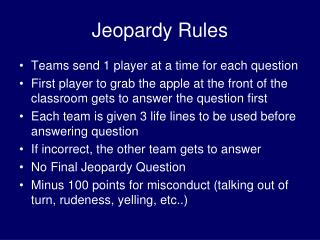Download PresentationJeopardy Rules

# Jeopardy Rules - PowerPoint PPT PresentationDownload Presentation## Jeopardy Rules

- - - - - - - - - - - - - - - - - - - - - - - - - - - E N D - - - - - - - - - - - - - - - - - - - - - - - - - - -
##### Presentation Transcript

1. Jeopardy Rules • Teams send 1 player at a time for each question • First player to grab the apple at the front of the classroom gets to answer the question first • Each team is given 3 life lines to be used before answering question • If incorrect, the other team gets to answer • No Final Jeopardy Question • Minus 100 points for misconduct (talking out of turn, rudeness, yelling, etc..)

2. A B C D E \$100 \$100 \$100 \$100 \$100 \$200 \$200 \$200 \$200 \$200 \$300 \$300 \$300 \$300 \$300 \$400 \$400 \$400 \$400 \$400 \$500 \$500 \$500 \$500 \$500

3. Marlon thinks he discovered a divisibility rule for 4. His rule states, “If you take an even number and multiply it by 100, then subtract the original number, the result is always divisible by 4. Which of the following numbers is a counterexample for Marlon’s rule?A) 2 B) 4C) 8 D) 12

4. Marlon thinks he discovered a divisibility rule for 4. His rule states, “If you take an even number and multiply it by 100, then subtract the original number, the result is always divisible by 4. Which of the following numbers is a counterexample for Marlon’s rule?A) 2

5. Nick said, “I’ve observed that when I flip a coin, I am equally likely to get heads or tails. So I predict that if I flip a coin four more times, I will get 2 heads, and 2 tails.”Nicole said, “The laws of probability state that for a balanced coin, either outcome is equally likely. So if I flip a coin four more times, I will get 2 heads and 2 tails.”Which of these describes their reasoning?A) Nick used inductive reasoning. Nicole used deductive reasoningB) They both used deductive reasoning.C) They both used inductive reasoning.D) Nick used deductive reasoning. Nicole used inductive reasoning.

6. Nick said, “I’ve OBSERVED that when I flip a coin, I am equally likely to get heads or tails. So I predict that if I flip a coin four more times, I will get 2 heads, and 2 tails.”Nicole said, “The laws of probability state that for a balanced coin, either outcome is equally likely. So if I flip a coin four more times, I will get 2 heads and 2 tails.”Which of these describes their reasoning?A) Nick used inductive reasoning. Nicole used deductive reasoning

7. In the figure, ∆ACD ~ ∆BCA. What is the length of AC? A) 4 B) 5 C) 16 D) 20

8. What do the letters CPCTC stand for?

9. What do the letters CPCTC stand for?Corresponding Parts of Congruent Triangles are Congruent

10. Johnny has two pencils. One is 8 cm long, and the other is 17 cm long. He then finds a third pencil that he wants to use to form a triangle with the other two. Which of the following pencils will allow him to form a triangle? • 4 cm long pencil • 9 cm long pencil • 22 cm long pencil • D) 25 cm long pencil

11. Johnny has two pencils. One is 8 cm long, and the other is 17 cm long. He then finds a third pencil that he wants to use to form a triangle with the other two. Which of the following pencils will allow him to form a triangle? C) 22 cm long pencil

12. Tim is painting a wall shaped like a trapezoid, as shown. What is the area of the wall?

13. Tim is painting a wall shaped like a trapezoid, as shown. What is the area of the wall? 330 ft²

14. What is the measure of an interior angle of a hexagon?

15. Find the area of a rhombus with diagonal lengths of • 10 inches and 14 inches. • 35 square inches • 70 square inches • 140 square inches • 280 square inches

16. Find the area of a rhombus with diagonal lengths of • 10 inches and 14 inches. • 70 square inches

17. Find the height of an equilateral triangle, with sides of length 24. Height = 12√3

18. Reflect point A (-4, 6) across the x-axis. What is A’ ?

19. Given that AB is parallel to CD and BC is parallel to AD, which angle is congruent to ∠CBD? A) ∠ABD B) ∠BAD C) ∠BDC D) ∠BDA

20. Given that AB is parallel to CD and BC is parallel to AD, which angle is congruent to ∠CBD? D) ∠BDA

21. Rectangle ACDF has an area of 50 square feet. What is the relationship between the sum of the areas of ∠AEC, ∠FBD, ∠CGD with the area of rectangle ACDF?

22. Rectangle ACDF has an area of 50 square feet. What is the relationship between the sum of the areas of ∠AEC, ∠FBD, ∠CGD with the area of rectangle ACDF? The sum of the triangles’ areas is 1.5 times the area of the rectangle

23. Heptagon PQRSTUV is an outline of a camping tent. What is the measure of ∠P?

24. Heptagon PQRSTUV is an outline of a camping tent. What is the measure of ∠P? 70°

25. C 400

26. C 500

27. D 100

28. D 200

29. Daily Double

30. D 300

31. D 400

32. D 500

33. E 100

34. E 200

35. E 300